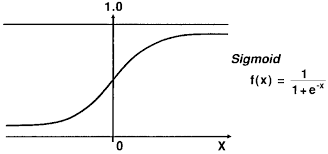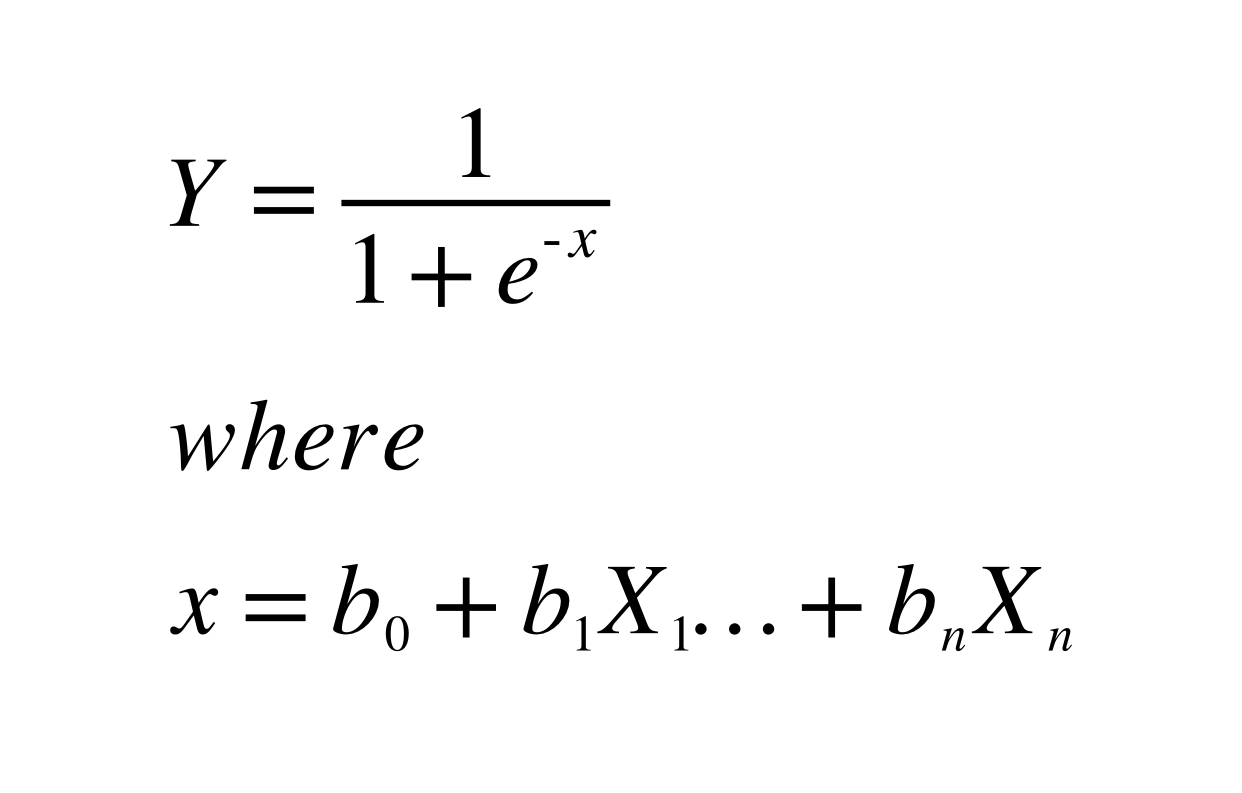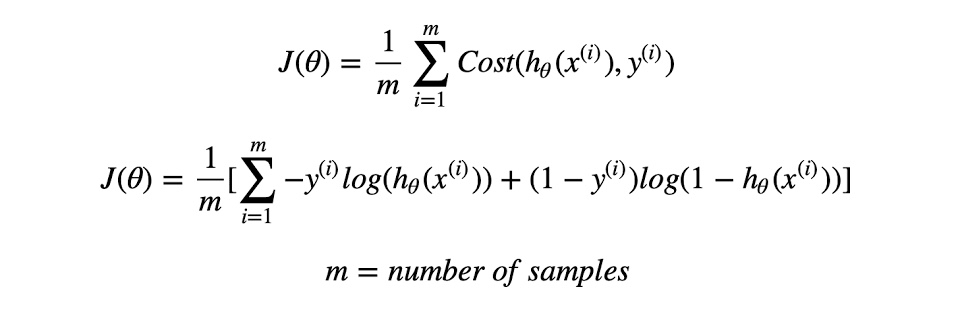# Beginning with ML 3.0: Logistic Regression

Sept. 28, 2020 395You can’t embark upon the Machine Learning journey and not encounter the term ‘Logistic Regression’. It’s one of the most easily understandable and commonly implemented algorithms! While Linear Regression (which I talk about https://www.codesdope.com/blog/author/71258/?author=71258 here, by the way) is used to predict a continuous dependent variable given a set of independent features, logistic regression is used for categorical classification. In other words, logistic regression can often be used when the question your algorithm is supposed to ask can be answered with a simple ‘yes’ or ‘no’. This is formally known as binomial logistic regression. (It can also be used to answer questions that have slightly more complex answers using multinomial logistic regression.) This algorithm has numerous applications in the real world. Logistic regression can be used to detect whether email is spam or not, to identify whether a tumor is malignant or not and even to detect whether a comment on one of your posts is sexist or not! In this article, we’ll walk through how logistic regression works with the help of a real world scenario.

Before that, let’s get an intuitive idea of our algorithm. This supervised algorithm was named after its core, the logistic function. Also known as the sigmoid function, the S shaped curve shown below takes values between 0 to 1 (exclusive at the both ends, meaning the resulting value will never be exactly 0 or 1) and forms the basis for logistic regression.As seen above, logistic regression (much like linear regression) uses an equation for its representation. Unlike linear regression, logistic regression predicts the probability of the first class (default class), instead of a numerical value. However, it was established earlier that we use this algorithm to answer yes or no questions. We must then ask, how can probability translate into yes or no? The answer is that logistic regression is a linear method that uses the sigmoid function for transformations. This means that the sigmoid function shown above is used to turn the output probabilities into binary (yes or no) output. This will become clear (and hopefully more intuitive) later on in this article.

Let’s discuss a real world example. Suppose a group of medical students are interested in studying preventive measures for heart attacks. Even without getting into the biology behind it, we know that there are multiple factors to consider while looking at the chances of having a heart attack, such as BMI (Body Mass Index), age and maybe even gender. Here, Logistic regression can be used to define a relationship between various factors, known as the predictor variables and the target variable, which in this case is the probability of a patient having a heart attack. For pure visualisation purposes, our sample dataset looks like this:

 Patient ID BMI Gender Age Had a Heart Attack A 27.3 Female 25 No B 24.5 Male 67 No C 28.2 Female 73 Yes D 27.9 Male 81 Yes

Obviously, a model would need much more data than this to be able to define the relationship between the predictor variables and the target variable. However, the fact remains that there is a relationship between our variables here. As humans, we can infer from the above table that age plays a very important role in this relationship- the older the patient, the more likely it is that they have a heart attack. We can also deduce that a high BMI is also indicative of higher chances of a heart attack. What we cannot deduce, is the precise relationship between these variables- i.e, at what age does a particular BMI indicate a very high chance of the patient suffering a heart attack? How does gender play into this? Even if we were shown a thousand more rows of data, we’d take a ridiculously long time to manually answer these questions accurately. But, lucky for us, our logistic regression model could probably answer this question with another thousand rows of data and it would do it pretty quickly too. The relationship between the predictor variables would then look like this:Here, X1 - Xn  represents the predictor variables (BMI, age and gender) and Y represents the target variable (heart attack). b0-bn  represent the parameters of the models, which signify the importance of each predictor variable. The parameters are determined by minimising the cost function with an optimisation algorithm like gradient descent. For Logistic Regression, the cost function looks like this:(Here, h(x) is equivalent to Y in our above figure and theta represents the parameter.) The equation has actually been designed very well and those of you who wish to understand it in depth (believe me, it’s not as scary as it looks) can look https://en.wikipedia.org/wiki/Logistic_regression here.

Now, for every patient in the dataset, Y is a probabilistic value that lies between 0 and 1. However, we require a straight answer. For the purpose of this exercise, we don’t want to conclude that there is a 0.65 chance that patient C had a heart attack. We want our model to tell us that C had a heart attack. Because we want to be able to use this model to tell a new patient, “We have a model that’s p% accurate and it does/doesn’t indicate that you’re at risk of a heart attack.” This is why the logistic regression model has a threshold value, usually set at 0.5. This implies that if Y= 0.65 for Patient C, the Y we see is 1. This transformation is done by the sigmoid function. Similarly, Y=0.37 is reported as 0, Y=0.96 is reported as 1 and so on. In this analogy, 1 corresponds to ‘yes’ and 0 to ‘no’.

Lastly, let’s also briefly discuss the p% accuracy that was mentioned above. How do we evaluate accuracy for a model like this? Well, there are different ways to evaluate a model, but when we talk about accuracy, we talk about the average number of correct predictions. This means that if our trained model predicts eighty outcomes correctly out of a hundred, its accuracy is 80%.

That’s about it! Hopefully, you have a clear understanding of not only the basic math behind logistic regression, but also it’s working and applications. Stay tuned for my next article which will focus on another popular supervised algorithm, the Naive Bayes algorithm! :)

Liked the post?
I'm an engineer and writer, passionate about Artificial Intelligence. My long term goal is to use NLP and ML to create sustainable algorithms that are capable of making logical decisions, thereby contributing to the betterment of society. Looking to change the world with a combination of innovation and kindness.
0 COMMENT